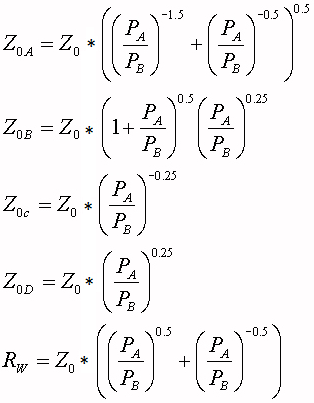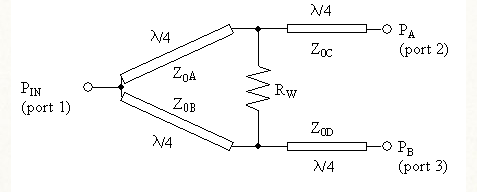# Wilkinson Power Divider Calculator

This CalcTown calculator calculates the parameters related to Wilkinson's power-divider circuit.

In the field of microwave engineering and circuit design, the Wilkinson Power Divider is a specific class of power divider circuit that can achieve isolation between the output ports while maintaining a matched condition on all ports. The Wilkinson design can also be used as a power combiner because it is made up of passive components and hence reciprocal. First published by Ernest J. Wilkinson in 1960, this circuit finds wide use in radio frequency communication systems utilizing multiple channels since the high degree of isolation between the output ports prevents cross-talk between the individual channels.

It uses quarter wave transformers, which can be easily fabricated as quarter wave lines on printed circuit boards. It is also possible to use other forms of transmission line (e.g. coaxial cable) or lumped circuit elements (inductors and capacitors).

It splits an input signal into two equal phase output signals, or combines two equal-phase signal into one in the opposite direction. Wilkinson relied on quarter-wave transformers to match the split ports to the common port.

#### Result

Ω
Ω
Ω
Ω
ΩWhere,

PA = Power output of Port 2

PB = Power output of Port 3

Z0 = Characteristic Impedance of the overall system

Rw = Terminating resistance between two output ports.

Z0A, Z0B, Z0C, Z0D = Branch impedances

Network theorem governs that a divider cannot satisfy all three conditions (being matched, reciprocal and loss-less) at the same time. Wilkinson divider satisfies the first two (matched and reciprocal), and cannot satisfy the last one (being loss-less). Hence, there is some loss occurring in the network.

No loss occurs when the signals at ports 2 and 3 are in phase and have equal magnitude.

However, some modification can be done to achieve unequal power division at the output ports. By cascading, the input power might be divided to any n-number of outputs.